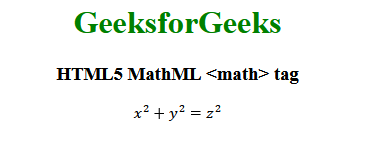Related Articles

# HTML5 | MathML <math> tag

• Last Updated : 05 Nov, 2019

The MathML <math> tag in HTML5 is the most prioritize element. Whatever MathML element you want to use they should wrapped inside of the <math> tag.

Syntax:

`[itex] child elements [/itex]`

Attributes: The tag accepts some attributes which are listed below:

• class|id|style: This attribute is used to hold the styles of the child elements.
• dir: This attributes holds the direction value. It holds two types of direction values ltr for left to right and rtl for right to left.
• href: This attribute is used to hold any hyperlink to a specified URL.
• mathbackground: This attribute holds the value of the math expressions background color.
• mathcolor: This attribute holds the color of the math expressions.
• display: This attribute holds the value of rendering of HTML element. There can be two value block which means that this element will be displayed outside the current span of text, inline which means that this element will be displayed inside the current span of text.
• mode: Express the display attribute, possible values are display and inline.
• overflow: It holds the value how the expression will behave. The default value is the linebreak and other possible values are scroll, elide, truncate, and scale.

Below example illustrates the MathML <math> tag in HTML5:

Example:

 ```<``html``>``  ` `<``head``>``    ``<``title``>HTML5 MathML math tag````  ` `<``body``>``    ``<``center``>``        ``<``h1` `style``=``"color:green"``>``            ``GeeksforGeeks``        ````         ` `        ``<``h3``>HTML5 MathML <``math``> tag``         ` `        ``<``math``>``            ``<``mrow``>``                ``<``mrow``>``                    ``<``msup``>``                        ``<``mi``>x``                        ``<``mn``>2``                    ````                    ``<``mo``>+``                    ``<``msup``>``                        ``<``mi``>y``                        ``<``mn``>2``                    ````                ````                ``<``mo``>=``                ``<``msup``>``                    ``<``mi``>z``                    ``<``mn``>2``                ````            ````        ````    ``````  ` ``

Output:Supported Browsers: The browsers supported by HTML5 MathML <math> tag are listed below:

• Firefox

Attention reader! Don’t stop learning now. Get hold of all the important Comcompetitivepetitve Programming concepts with the Web Design for Beginners | HTML  course.

My Personal Notes arrow_drop_up